ODS Graphics

Statistical procedures use ODS Graphics to create graphs as part of their output. ODS Graphics is described in detail in Chapter 21, Statistical Graphics Using ODS.

Before you create graphs, ODS Graphics must be enabled (for example, with the ODS GRAPHICS ON statement). For more information about enabling and disabling ODS Graphics, see the section Enabling and Disabling ODS Graphics in Chapter 21, Statistical Graphics Using ODS.

The overall appearance of graphs is controlled by ODS styles. Styles and other aspects of using ODS Graphics are discussed in the section A Primer on ODS Statistical Graphics in Chapter 21, Statistical Graphics Using ODS.

### ODS Graph Names

PROC KDE assigns a name to each graph it creates using the Output Delivery System (ODS). You can use these names to reference the graphs when using ODS. The names are listed in Table 47.2.

Table 47.2 Graphs Produced by PROC KDE

ODS Graph Name

Plot Description

Statement

PLOTS= Option

BivariateHistogram

Bivariate histogram of data

BIVAR

HISTOGRAM

ContourPlot

Contour plot of bivariate kernel density estimate

BIVAR

CONTOUR

ContourScatterPlot

Contour plot of bivariate kernel density estimate overlaid with scatter plot

BIVAR

CONTOURSCATTER

DensityPlot

Univariate kernel density estimate curve

UNIVAR

DENSITY

DensityOverlayPlot

Overlaid univariate kernel density estimate curves

UNIVAR

DENSITYOVERLAY

HistogramDensity

Univariate histogram overlaid with kernel density estimate curve

UNIVAR

HISTDENSITY

Histogram

Univariate histogram of data

UNIVAR

HISTOGRAM

HistogramSurface

Bivariate histogram overlaid with surface plot of bivariate kernel density estimate

BIVAR

HISTSURFACE

ScatterPlot

Scatter plot of data

BIVAR

SCATTER

SurfacePlot

Surface plot of bivariate kernel density estimate

BIVAR

SURFACE

### Bivariate Plots

You can specify the PLOTS= option in the BIVAR statement to request graphical displays of bivariate kernel density estimates.

PLOTS= option1 <option2 ...>

requests one or more plots of the bivariate kernel density estimate. The following table shows the available plot options.

 Option Description ALL all available displays CONTOUR contour plot of bivariate density estimate CONTOURSCATTER contour plot of bivariate density estimate overlaid with scatter plot of data HISTOGRAM bivariate histogram of data HISTSURFACE bivariate histogram overlaid with bivariate kernel density estimate NONE suppresses all plots SCATTER scatter plot of data SURFACE surface plot of bivariate kernel density estimate

By default, if ODS Graphics is enabled and you do not specify the PLOTS= option, then the BIVAR statement creates a contour plot. If you specify the PLOTS= option, you get only the requested plots.

### Univariate Plots

You can specify the PLOTS= option in the UNIVAR statement to request graphical displays of univariate kernel density estimates.

PLOTS= option1 <option2 ...>

requests one or more plots of the univariate kernel density estimate. The following table shows the available plot options.

 Option Description ALL all available displays DENSITY univariate kernel density estimate curve DENSITYOVERLAY overlaid univariate kernel density estimate curves HISTDENSITY univariate histogram of data overlaid with kernel density estimate curve HISTOGRAM univariate histogram of data NONE suppresses all plots

By default, if ODS Graphics is enabled and you do not specify the PLOTS= option, then the UNIVAR statement creates a histogram overlaid with a kernel density estimate. If you specify the PLOTS= option, you get only the requested plots.

### Binning of Bivariate Histogram

Let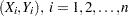, be a sample of sizedrawn from a bivariate distribution. For the marginal distribution of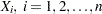, the number of bins (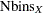) in the bivariate histogram is calculated according to the formula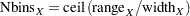where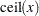denotes the smallest integer greater than or equal to,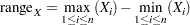and the optimal bin width is obtained, following Scott (1992, p. 84), as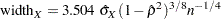Here,
and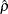are the sample variance and the sample correlation coefficient, respectively. When you specify a WEIGHT variable, PROC KDE uses weighted versions ofandin the preceding expressions.

Similar formulas are used to compute the number of bins for the marginal distribution of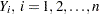. Further details can be found in Scott (1992).

Notice that if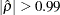, thenis calculated as in the univariate case (see Terrell and Scott; 1985). In this case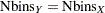.